# Introduction to Integration

Integration is a way of adding slices to find the whole.

Integration can be used to find areas, volumes, central points and many useful things. But it is easiest to start with finding the area under the curve of a function like this:What is the area under y = f(x) ?

## Slices

 We could calculate the function at a few points and add up slices of width Δx like this (but the answer won't be very accurate):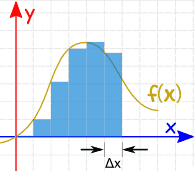We can make Δx a lot smaller and add up many small slices (answer is getting better):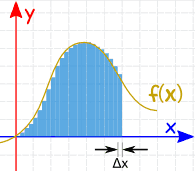And as the slices approach zero in width, the answer approaches the true answer. We now write dx to mean the Δx slices are approaching zero in width.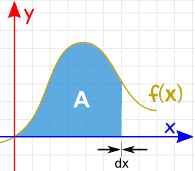## That is a lot of adding up!

But we don't have to add them up, as there is a "shortcut". Because ...

... finding an Integral is the reverse of finding a Derivative.

Like here:

### Example: What is an integral of 2x?We know that the derivative of x2 is 2x ...

... so an integral of 2x is x2

You will see more examples later.

## Notation

 The symbol for "Integral" is a stylish "S" (for "Sum", the idea of summing slices):After the Integral Symbol we put the function we want to find the integral of (called the Integrand),

and then finish with dx to mean the slices go in the x direction (and approach zero in width).

And here is how we write the answer: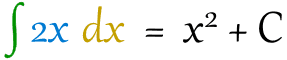## Plus C

We wrote the answer as x2 but why + C ?

It is the "Constant of Integration". It is there because of all the functions whose derivative is 2x:The derivative of x2+4 is 2x, and the derivative of x2+99 is also 2x, and so on! Because the derivative of a constant is zero.

So when we reverse the operation (to find the integral) we only know 2x, but there could have been a constant of any value.

So we wrap up the idea by just writing + C at the end.

## Tap and TankIntegration is like filling a tank from a tap.

The input (before integration) is the flow rate from the tap.

Integrating the flow (adding up all the little bits of water) gives us the volume of water in the tank.

### Simple Example: Constant Flow RateIntegration: With a flow rate of 1, the tank volume increases by x

Derivative: If the tank volume increases by x, then the flow rate is 1

This shows that integrals and derivatives are opposites!

### Now For An Increasing Flow Rate

Imagine the flow starts at 0 and gradually increases (maybe a motor is slowly opening the tap).As the flow rate increases, the tank fills up faster and faster.

Integration: With a flow rate of 2x, the tank volume increases by x2

Derivative: If the tank volume increases by x2, then the flow rate must be 2x

### Example: with the flow in liters per minute, and the tank starting at 0

After 3 minutes (x=3):

• the flow rate has reached 2x = 2×3 = 6 liters/min,
• and the volume has reached x2 = 32 = 9 liters

And after 4 minutes (x=4):

• the flow rate has reached 2x = 2×4 = 8 liters/min,
• and the volume has reached x2 = 42 = 16 liters

We can do the reverse, too:Imagine you don't know the flow rate.
You only know the volume is increasing by x2.

We can go in reverse (using the derivative, which gives us the slope) and find that the flow rate is 2x.

### Example:

• At 1 minute the volume is increasing at 2 liters/minute (the slope of the volume is 2)
• At 2 minutes the volume is increasing at 4 liters/minute (the slope of the volume is 4)
• At 3 minutes the volume is increasing at 6 liters/minute (a slope of 6)
• etcSo Integral and Derivative are opposites.

We can write that down this way:

 The integral of the flow rate 2x tells us the volume of water: ∫2x dx = x2 + C And the slope of the volume increase x2+C gives us back the flow rate:(x2 + C) = 2xAnd hey, we even get a nice explanation of that "C" value ... maybe the tank already has water in it!

• The flow still increases the volume by the same amount
• And the increase in volume can give us back the flow rate.

Which teaches us to always add "+ C".

## Other functions

Well, we have played with y=2x enough now, so how do we integrate other functions?

If we are lucky enough to find the function on the result side of a derivative, then (knowing that derivatives and integrals are opposites) we have an answer. But remember to add C.

### Example: what is ∫cos(x) dx ?From the Rules of Derivatives table we see the derivative of sin(x) is cos(x) so:

cos(x) dx = sin(x) + C

But a lot of this "reversing" has already been done (see Rules of Integration).

### Example: What is ∫x3 dx ?

On Rules of Integration there is a "Power Rule" that says:

xn dx = xn+1n+1 + C

We can use that rule with n=3:

x3 dx = x44 + C

Knowing how to use those rules is the key to being good at Integration.

So get to know those rules and get lots of practice.

Learn the Rules of Integration and Practice! Practice! Practice!
(there are some questions below to get you started)

## Definite vs Indefinite Integrals

We have been doing Indefinite Integrals so far.

A Definite Integral has actual values to calculate between (they are put at the bottom and top of the "S"):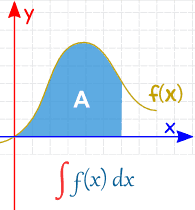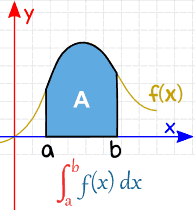Indefinite Integral Definite Integral